# Convergence of Random Variable

When we talk about convergence of random variable, we want to study the behavior of a sequence of random variables {Xn}=X1, X2,…,Xn,… when n tends towards infinity. Basically, we want to give a meaning to the writing:

A sequence of random variables, generally speaking, can converge to either another random variable or a constant.

However, there are three different situations we have to take into account:

• Convergence in Probability

--

--

--

## More from DataSeries

Imagine the future of data

## Achieving Mathematical Maturity## A Brief History of Volatility Models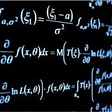## The Perfect Matching: Part 1## What is discrete mathematics??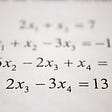## NBA playoff win chances via Bayesian inference## Linear Algebra 101 — Part 1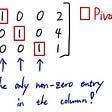## Probability theory: Multivariate random variable, the distribution function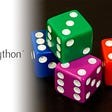## Valentina Alto

Cloud Specialist at @Microsoft | MSc in Data Science | Machine Learning, Statistics and Running enthusiast

## Robust Statistics: The Influence Function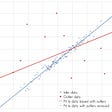## Types of probability distributions## Interpretations of Probability## Bayes’ Theorem Theoretical Explanation With Example